# Parallel Circuits: Calculating Currents with Ohm's Law

Lesson Transcript
Instructor: Matthew Bergstresser

Matthew has a Master of Arts degree in Physics Education. He has taught high school chemistry and physics for 14 years.

In parallel circuits, current has more than one path to take. Whenever it comes to a junction, it either splits into multiple currents or combines into one current. In this lesson, we will learn how to determine current in loops of parallel circuits.

## Opening Door Example

Imagine five people walking single-file through a hallway and they come to two doors leading to two different hallways. If two people go through door A, how many go through door B? The answer is 3. At the end of the hallways, there are two more doors which lead to another hallway. How many people enter the final hallway? All five people! This is an analogy of how current splits and recombines at junctions in parallel circuits. Let's look at how we can mathematically calculate the amount of current in each path.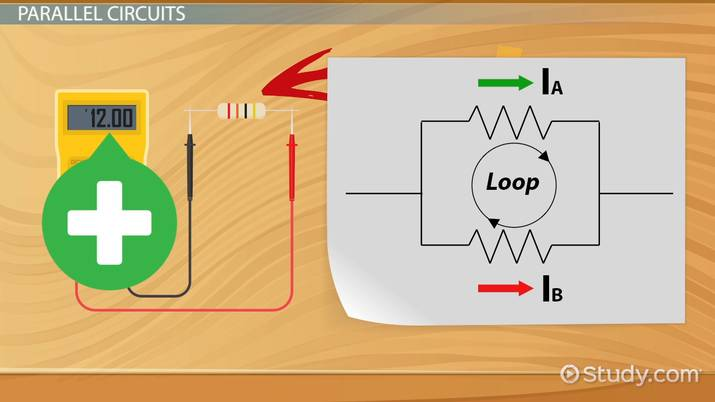An error occurred trying to load this video.

Try refreshing the page, or contact customer support.

Coming up next: Ohm's Law Formula & Circuit Calculation Practice

### You're on a roll. Keep up the good work!

Replay
Your next lesson will play in 10 seconds
• 0:00 Opening Door Example
• 0:37 Parallel Circuits
• 4:42 Lesson Summary
Save Save

Want to watch this again later?

Timeline
Autoplay
Autoplay
Speed Speed

## Parallel Circuits

A parallel circuit is one where current splits and recombines at junctions. An example of a parallel circuit is shown in the diagram on your screen below: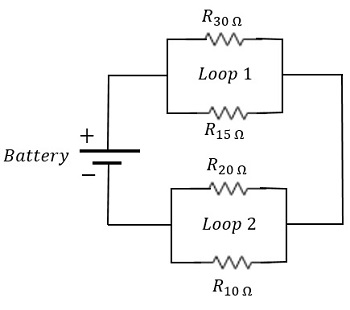Current leaves the positive terminal of the battery heading for Loop 1. In this next diagram on your screen, the junctions where current splits are circled in green, and where the currents combine into one are circled in red.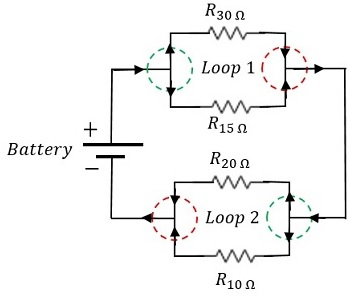Kirchhoff's junction rule is an application of the conservation of electrical charge, and states that current into a junction must equal current out of the junction. Ohm's law states that voltage equals the product of current in amps and resistance in ohms, which can be written as: V = IR.

Kirchhoff's loop rule sums all of the voltages in a closed loop and sets them equal to zero. There's no potential difference at a single point in a circuit. It's like saying there is no change in elevation at a single point on a mountain. We use Ohm's law (IR) to determine the voltages across each resistor. If the loop is in the same direction as the current, the voltage across the resistor is negative. If the loop is in the opposite direction as the current, the voltage is positive.

Let's work an example where we have to determine the currents in the loops of a parallel circuit. Let's take a look at an example.

Here's a prompt: Determine the currents through all of the resistors in the parallel circuit shown here in this third diagram you're looking at on your screen below: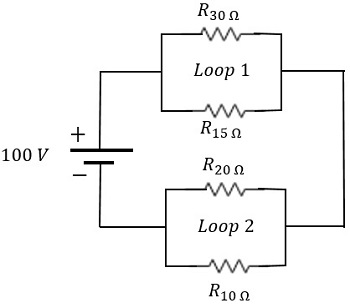Solution: We'll start with Kirchhoff's junction rule at the left junction in Loop 1, and the right junction in Loop 2.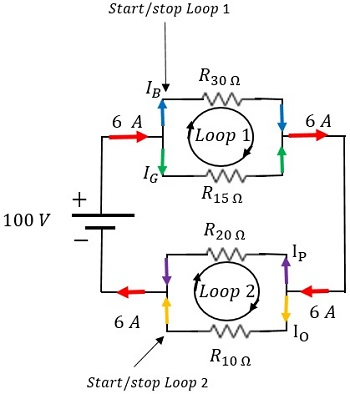Notice the current entering and leaving Loop 1 is 6 amps, which is the same current entering and leaving Loop 2.

This chart shows the two equations generated from Kirchhoff's junction rule.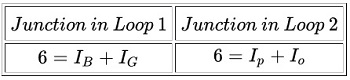Since we have four unknowns, we need four equations, and we already have two. The chart on your screen below shows the other two equations that come from Kirchhoff's loop rule for Loops 1 and 2.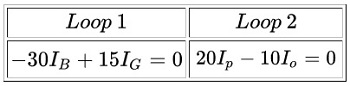Remember, when the current is in the same direction as the loop, the voltage is negative. When the current is in the opposite direction of the loop, the voltage is positive.

To unlock this lesson you must be a Study.com Member.

## Practice:Parallel Circuits: Calculating Currents with Ohm's Law Quiz

1/5 completed

### If I1 = 2 A, and I2 = 3 A, what is I3?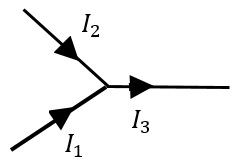Create Your Account To Take This Quiz

As a member, you'll also get unlimited access to over 84,000 lessons in math, English, science, history, and more. Plus, get practice tests, quizzes, and personalized coaching to help you succeed.

It only takes a few minutes to setup and you can cancel any time.

### Register to view this lesson

Are you a student or a teacher?